﻿ Investigation on Tri-hexagonal Boron Nanotube by Exploiting the Certain Topological Indices and Their <i>M</i><i>-</i>polynomialsPublications are Open
Access in this journal
Article Versions
Export Article
• Normal Style
• MLA Style
• APA Style
• Chicago Style
Research Article
Open Access Peer-reviewed

### Investigation on Tri-hexagonal Boron Nanotube by Exploiting the Certain Topological Indices and Their M-polynomials

V. Lokesha, Sushmitha Jain , T. Deepika
Turkish Journal of Analysis and Number Theory. 2017, 5(6), 197-201. DOI: 10.12691/tjant-5-6-1
Received July 01, 2017; Revised August 15, 2017; Accepted September 21, 2017

### Abstract

Due to the presence of multicenter bonds and their novel electronic properties, boron nanotubes are attractive. The tri-hexagonal boron nanotubes are build up from triangles and hexagons. It is useful to the QSPR/QSAR studies. Topological indices are classified in different forms such as, degree based topological indices, distance based topological indices and counting related topological indices etc. Here, we concentrated the reckoning of topological indices such as first zagreb, second zagreb, modified second zagreb index, generalized randic index, symmetric division degree index for the tri-hexagonal boron nanotube. Also, established their M-polynomials and using maple software plotted the 3D structure.

### 1. Introduction

Chemical graph theory is an important branch of graph theory as it contains discrete Adriatic indices. In this theory, a descriptor of a molecular graph G is a number related to the structure of G and is invariant under the automorphisms of the graph.

Recently, D. Vukicevic 24 revealed the set of 148 discrete Adriatic indices. They were analyzed on the testing sets provided by the International Academy of Mathematical Chemistry (IAMC) and it had been shown that they have good predictive properties in many cases. There was a vast research regarding various properties of these topological indices.

There are two oldest degree based topological indices, first and second zagreb indices were introduced more than thirty years ago by Gutman and Trinajstic 11 which are defined as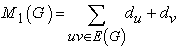(1)

and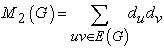(2)

where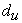denotes the degree of a vertex u in G.

Both the first zagreb index and the second zagreb index give greater weights to the inner vertices and edges, and smaller weights to the outer vertices and edges, which opposes intuitive reasoning.

For a simple connected graph G, the second modified zagreb index 18 is defined as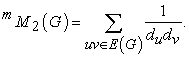(3)

Symmetric division deg index 8, 9 is one of the discrete Adriatic indices that is good predictor of total surface area for polychlorobiphenyls. The symmetric division degree index of a connected graph G is defined as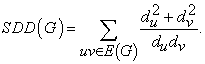(4)

The Randi´c index 19 also the most popular, the most often applied and the most studied among all other topological indices. The Randi´c index denoted by R(G) and introduced by Milan Randi´c in 1975, is also one of the oldest topological index.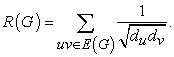(5)

In 1998, working independently, Bollobas and Erdos 2 and Amic et. al., 1 proposed the generalised Randi´c index and has been studied extensively by both chemists and mathematicians. The ordinary Randi´c connectivity index has been extended to the general Randi´c connectivity index defined as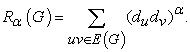(6)

The inverse sum index (ISI) is a significant predictor of total surface area for octane isomers these are introduced by Damir Vukicevic and Marija Gasperov 4.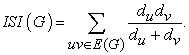(7)

Another variant of the Randi´c index is the harmonic index 21 defined as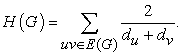(8)

The M-polynomial 14 of graph G is defined as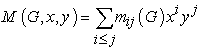(9)

where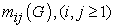be the number of edges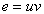of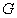such that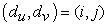.

Where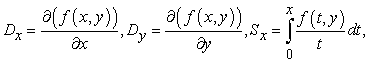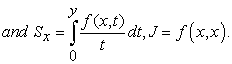### 2. Tri-hexagonal Boron Nanotube

In 1991 nanotubes were discovery, it has created intense experimental and theoretical interest in such structures. Theoretical perspectives of carbon tubes suggest that their electrical properties will range from metallic to semi-conducting, depending on the tube diameter.

In 2004 the first boron triangular nanotubes were innovated and evolved from a triangular sheet. The latest discovery of boron triangular nanotubes challenges the monopoly of carbon nanotubes (CNTs). Researchers believe that carbon nanotubes are less significant than boron triangular nanotubes. Eventually scientists have proved this speculation by innovating the world’s smallest superconductor using nanoscale molecular superconducting boron wires 3. As for as special features are concerned boron nanotubes also have some better features when compared to CNTs such as high chemical stability, high resistance to oxidation at high temperatures and are a stable wide band-gap semiconductor. Because of these properties, they can be used for applications at high temperatures or in corrosive environments such as batteries, fuel cells, super capacitors, high speed machines as solid lubricant.

• Figure 1. Three-dimensional perception of tri-hexagonal boron nanotube

The boron triangular nanotubes are evolved from CNTs by adopting an additional atom to the center of each hexagon. A new class of boron nanotubes which are constructed from triangles and hexagons, called the tri-hexagonal boron nanotube. These nanotubes are formed by removing some atoms from boron triangular nanotubes.

These nanotubes are sparser than the other boron nanotube and after relaxation it remains at and metallic independent of their chirality.

We denote this nanotube by C3C6(H)[m; n], where m is the number of hexagons in one column and n is the number of hexagons in one row in two-dimensional lattice of C3C6(H)[m; n] nanotube.

• Figure 2. The graph of C3C6(H)[m; n] nanotube

Recently, I. Nadeem, H. Shaker 13 obtained the expressions for certain topological indices of tri-hexagonal boron nanotube. This motivates us to reckon on certain topological indices for the tri-hexagonal boron nanotube and structured their corresponding M-polynomials. Finally, Wrapping up 3D plot of concerned Topological indices utilized for Maple Software.

### 3. Main Results

In this section, we present our main results. In the following, we determine the topological indices and M-polynomials of the tri-hexagonal boron nanotube.

Theorem 1. Consider the tri-hexagonal boron nanotube T = C3C6 (H) [m; n], then

1.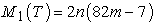2.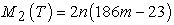3.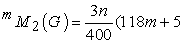4.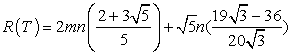5.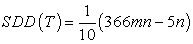6.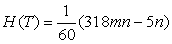7.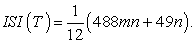Proof. Let the total number of vertices in tri hexagonal boron nanotube is 8mn and n(18m-1) number of edges.

Now, if we partition the edges correspond to their degree of end vertices which are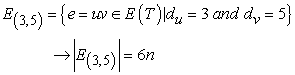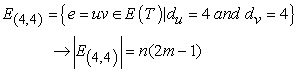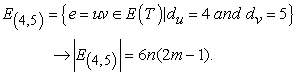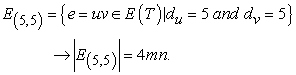• Figure 3. The graph of C3C6(H)[m; n] nanotube with m = 2 and n = 5

The representatives of these partite sets are shown in Figure 3 in which red, green, black and blue edges are edges belong to E(3;5), E(4;4), E(4;5) and E(5;5) respectively.

Now consider,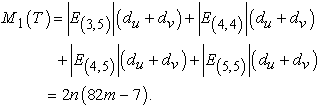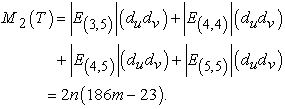Similarly, the proof is followed by the same technique by taking into account of edge partition and then applying Equations (3)-(8) to T = C3C6(H)[m; n], we come to the results as required.

Theorem 2. Let T be tri-hexagonal boron nanotube, then M-polynomial is

1.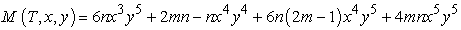2.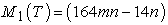3.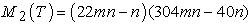4.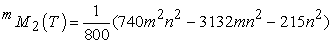5.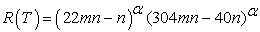6.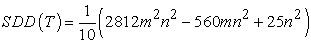7.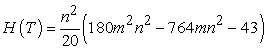8.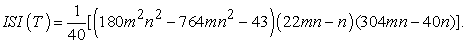Proof:

Let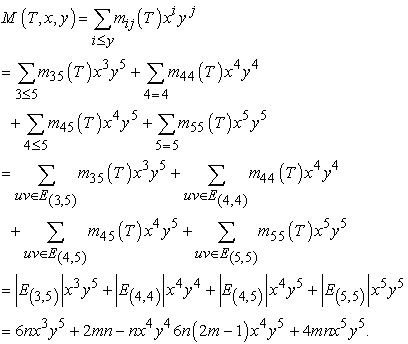Now, from M-polynomial equation we compute the following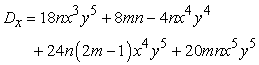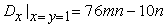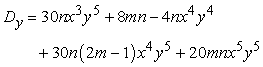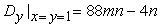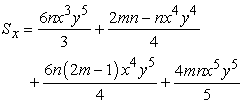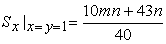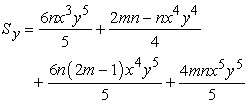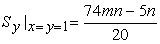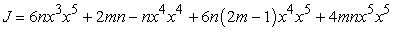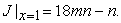Using Table 1 derivations of M-polynomial and the values of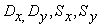and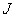we get the required results.

• Figure 4. The graph of M - polynomial of tri-hexagonal boron nanotube

### 4. Conclusion

In this paper, we computed the certain degree-based topological indices and M-polynomial. Also, plotted the 3D structure for tri-hexagonal boron nanotube. The topological indices calculated in here can help us to understand the physical features, chemical reactivity, and biological activities. In this perspective, topological index is considered as vital role that maps each molecular structure to a real number and is used as a descriptor of the molecule under testing.

### Acknowledgements

The second author is thankful to Maulana Azad National Fellowship, Ref.No.F117.1/201718/MANF201718KAR76148. First and third authors were thankful to Department of Science and Technology for research funding, Project No. SR/WOS-A/PM-43/2016.

### References

Published with license by Science and Education Publishing, Copyright © 2017 V. Lokesha, Sushmitha Jain and T. DeepikaThis work is licensed under a Creative Commons Attribution 4.0 International License. To view a copy of this license, visit http://creativecommons.org/licenses/by/4.0/